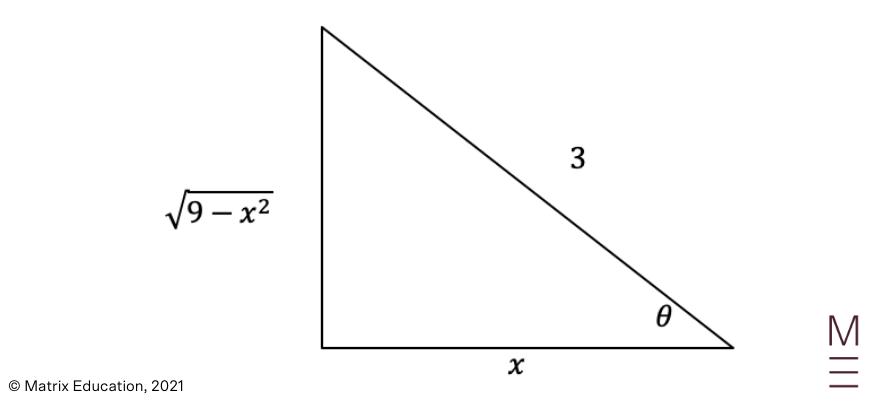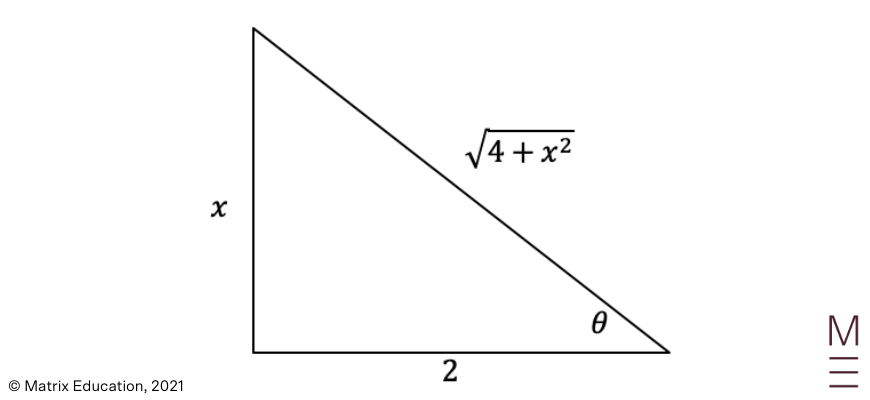# Part 7: Integration by Substitution | Beginner’s Guide to Year 12 Maths Ext 1

Integration by substitution is a crucial skill, read this article to build the right foundation for extension 1 and 2 Maths.Integration by substitution is a crucial skill for extension 1 maths and higher. There’s no substituteIntegration for mastering this! that’s why in this article, we give you a detailed overview and show you the key techniques and provide you with practice questions to test yourself with!

## Year 12 Mathematics Extension 1: Integration by Substitution

Understanding how to solve integrals using the method of substitution is an extremely important skill to master, as it enables students to solve harder integration questions, as well as further Calculus questions such as finding the area under curves or the volume of solids of revolution. Let’s now look at the NESA syllabus dot point for this topic!

### NESA Syllabus Outcome

• Find and evaluate indefinite and definite integrals using the method of integration by substitution, using a given substitution of:
• Change an integrand into an appropriate form using algebra.

## Assumed knowledge

Students should already be familiar with basic integration rules for linear, trigonometric, exponential and logarithmic functions, solving definite and indefinite integrals, trigonometric identities, as well as exponential and logarithmic laws.

## Linear Substitution

• We change the variable in a definite/indefinite integral to convert the integrand into a function that we know how to integrate.
• Follow these steps to change the variable:
1. Use a substitution to convert $$\int f(x) \ dx$$ to a simpler integral of a new variable $$u$$. Remember to convert $$dx$$ to $$du$$.
2. Simplify the integral.
3. Complete the integration process.
4. Revert the integrand to a function of $$x$$.

### Example 1:

Find $$\int x(x^2-4)^2 \ dx$$ using the substitution $$u=x^2-4$$.

### Solution:

 Steps Working out 1 Convert $$f(x)$$ into an integral with new variable $$u$$Given $$u=x^2-4$$\begin{align*} ∴\frac{du}{dx} &= 2x\\ ∴dx &= \frac{du}{2x}\\ \end{align*} 2 Simplify the integral\begin{align*} ∴ & \int x(x^2-4)^2 \ dx\\ = & \int u^2 \times x \times \frac{du}{2x}\\ = & \int \frac{1}{2} u^2 \ du\\ \end{align*} 3 Complete the integration processFrom the above integral, we get:\begin{align*} &= \frac{1}{2} \times \frac{u^3}{3} + C\\ &= \frac{1}{6}u^3 +C\\ \end{align*} 4 Revert the integrand back to a function of xAs $$u=x^2-4$$, we have: $$= \frac{1}{6} (x^2-4)^3+C$$i.e. $$\int x(x^2-4)^2 \ dx = \frac{1}{6} (x^2-4)^3 +C$$

For definite integrals, we also need to change the limits of the integral at the same time that the variable is changed. Refer to the following example on how to change the limits of the integral.

### Example 2:

Find the definite integral using the substitution .

### Solution:

 Steps Working out 1 Convert $$f(x)$$ into an integral with new variable $$u$$Given $$u=1+x$$\begin{align*} ∴\frac{du}{dx} &= 1\\ ∴dx &= du\\ \end{align*} 2 Change the limits of the integral using the given substitutionWhen $$x=0$$, $$u=1+0=1$$.When $$x=3$$, $$u=1+3=4$$. 3 Replace all the $$x$$‘s with $$u$$‘s in the integrand\begin{align*} \int_0^3 x(1+x)^2 \ dx &= \int_1^4 (u-1)u^2 \ du\\ &= \int_1^4u^2(u-1) \ du\\ \end{align*} 4 Integrate and evaluate. Since this is a definite integral, we no longer need to revert $$u$$‘s to $$x$$‘s\begin{align*} & \int_1^4 u^2(u-1) \ du\\ &= \int_1^4 u^3-u^2 \ du\\ &= \left[ \frac{u^4}{4}- \frac{u^3}{3} \right]_1^4\\ &= \left( \frac{4^4}{4} – \frac{4^3}{3} \right) – \left( \frac{1^4}{4} – \frac{1^3}{3} \right)\\ &= \frac{171}{4}\\ \end{align*}

## Need more practice with Integration by Substitution?## Trigonometric Substitution

Another integration by substitution technique is trigonometric substitution. Sometimes, we have functions that involve quadratics inside square roots or powers of square roots, such as $$\sqrt{9-x^2}$$ or $$(4+x^2)^{\frac{3}{2}}$$. We are unable to solve these functions via elementary integration methods, so hence a substitution is required. In order to implement an appropriate substitution, let us revise some trigonometric identities.

### Revision of trigonometric identities

From year 11, we know a very standard trigonometric identity which is,

$$sin^2(θ)+cos^2(θ)=1 \ \ \ \ (1)$$

With this identity, we can do some rearrangements and let

\begin{align*}
sin^2(θ) &= 1-cos^2(θ) \ \ \ \ \ (2)\\
cos^2(θ) &= 1 – sin^2(θ) \ \ \ \ \ \ \ (3)\\
\end{align*}

If we divide equation (1) by $$cos^2(θ)$$ we obtain another trigonometric identity, i.e.

$$tan^2(θ)+1=sec^2(θ)$$

Likewise, if we divide equation (1) by $$sin^2(θ)$$, we obtain our third trigonometric identity, i.e.

$$1+cot^2(θ)=cosec^2(θ)$$

In addition to those trigonometric identities, we should also brush up on our trigonometric derivatives to proceed with our trigonometric substitution technique.

### Revision of trigonometric derivatives

Recall the following derivatives of these trigonometric functions:

\begin{align*}
\frac{d}{dθ} (sin(θ)) &= cos(θ)\\
\frac{d}{dθ} (cos(θ)) &=-sin(θ)\\
\frac{d}{dθ} (tan(θ)) &= sec^2(θ)\\
\frac{d}{dθ} (cot(θ)) &= -cosec^2(θ)\\
\frac{d}{dθ} (sec(θ)) &= sec(θ)tan(θ)\\
\frac{d}{dθ} (cosec(θ)) &= -cosec(θ)cot(θ)\\
\end{align*}

Our goal is to replace square roots of these quadratic expressions with trigonometric functions instead using the above identities along with these derivatives.

### Integration using trigonometric substitutions

Now we explore an integration question that would require substitution.

#### Example 1:

Integrate $$\int \sqrt{9=x^2} \ dx$$ using the substitution $$x=3sin(θ)$$

#### Solution:

We begin with the appropriate substitution. Let

\begin{align*}
x &= 3sin(θ)\\
dx &= 3cos(θ) \ dθ\\
\end{align*}

Hence, the integral can be rewritten as

 \begin{align*} \int \sqrt{9-x^2} \ dx &= \int \sqrt{9-(3sin(θ))^2} \times 3cos(θ) \ dθ \\ &= \int \sqrt{9-9sin^2(θ)} \times 3cos(θ) \ dθ \\ &= \int \sqrt{9(1-sin^2(θ)} \times 3cos(θ) \ dθ \\ &= \int 3cos(θ) \times 3cos(θ) \ dθ\\ &= 9 \int cos^2(θ) \ dθ\\ &= 9 \int \frac{1}{2}(1+cos(2θ)) \ dθ\\ &= \frac{9}{2} \int (1+cos(2θ)) \ dθ\\ &= \frac{9}{2} \left( θ + \frac{sin(2θ)}{2} \right) + C\\ \end{align*}where $$C$$ is a constant.

Now a lot of students stop here, which is wrong. Remember, we’re not done solving the integral, since the original integral is in terms of $$x$$ not $$θ$$. Hence, we must convert the answer back to $$x$$ using inverse trigonometric functions.

We know that $$x=3cos(θ)$$, which means that $$θ=cos^{-1} \left( \frac{x}{3} \right)$$. But how can we convert $$sin(2θ)$$ back into $$x$$?

We use the double angle formula $$sin(2θ)=2sin(θ)cos(θ)$$, and then proceed by drawing a triangle using the condition $$θ=cos^{-1} \left( \frac{x}{3} \right)$$.Therefore,

\begin{align*}
sin(2θ) &= 2sin(θ)cos(θ)\\
&= 2 \times \frac{ \sqrt{9-x^2}}{3} \times \frac{x}{3}\\
&= \frac{2x \sqrt{9-x^2}}{9}\\
\end{align*}

Hence, our overall integral is

 \begin{align*} \int \sqrt{9-x^2} \ dx &= \frac{9}{2} \left( cos^{-1} (\frac{x}{3})+ \frac{1}{2} \times \frac{2x \sqrt{9-x^2}}{9} \right)\\ &= \frac{9}{2} cos^{-1} \left( \frac{x}{3} \right)+ \frac{x \sqrt{9-x^2}}{2}+C\\ \end{align*}where $$C$$ is a constant.

#### Example 2:

Integrate $$\frac{1}{(4+x^2)^{\frac{3}{2}}} \ dx$$ using the substitution $$x=2tan(θ)$$

#### Solution:

Once again, we begin with the given substitution,

\begin{align*}
x &= 2tan(θ)\\
dx &= 2sec^2(θ)\\
\end{align*}

Therefore,

 \begin{align*} \int \frac{1}{(4+x^2)^{\frac{3}{2}}} \ dx &= \int \frac{2sec^2(θ)}{(4+(2tan(θ))^2)^{\frac{3}{2}}} \ dθ\\ &= \int \frac{2sec^2(θ)}{8(1+tan^(θ))^{\frac{3}{2}}} \ dθ\\ &= \frac{1}{4} \int \frac{sec^2(θ)}{(sec^2(θ))^{\frac{3}{2}}} \ dθ\\ &= \frac{1}{4} \int \frac{sec^2(θ)}{sec^3(θ)} \ dθ\\ &= \frac{1}{4} \int \frac{1}{sec(θ)} \ dθ\\ &= \frac{1}{4} \int cos(θ) \ dθ\\ &= \frac{1}{4} sin(θ) + C \\ \end{align*}where $$C$$ is a constant.

We once again employ the ‘triangle method’ in the previous example to convert our current answer into an answer in terms of $$x$$.Therefore,

$$sin(θ)= \frac{x}{\sqrt{4+x^2}}$$

Hence,

$$\int \frac{1}{(4+x^2)^{\frac{3}{2}}} dx = \frac{x}{4 \sqrt{4+x^2}} +C$$

where $$C$$ is a constant.

## Exponential & Logarithmic Substitution

We also can have functions that have exponential and logarithmic expressions built in. Hence, we use the following useful exponential and logarithmic derivatives as part of our solving process,

\begin{align*}
\frac{d}{dx} e^{f(x)} &= f'(x)e^{f(x)}\\
\frac{d}{dx} ln[f(x)] &= \frac{f'(x)}{f(x)}\\
\end{align*}

These derivatives can allow us to convert these kinds of integrals into much simpler and solvable forms.

### Example 1:

Integrate $$\int_0^{ln(2)} \frac{e^{3x}}{e^{3x}+6} dx$$ by using the substitution $$u=e^{3x}$$.

### Solution:

We start off once again by implementing the given substitution, i.e.

\begin{align*}
u &=e^{3x}\\
du &= 3e^{3x} \ dx\\
\end{align*}

Since we’ve used a substitution here, it is KEY that we do NOT forget to change the boundaries:

\begin{align*}
x=0 &⇒ u=e^0=1\\
x=ln(2) &⇒ u=e^{3ln(2)} = 8\\
\end{align*}

Hence, the given integral can be rewritten as

 \begin{align*} \int_0^{ln(2)} \frac{e^{3x}}{e^{3x}+6} dx &= \frac{1}{3} \int_0^{ln(2)} \frac{3e^{3x}}{e^{3x}+6} dx\\ &= \frac{1}{3} \int_1^8 \frac{du}{u+6} \\ &= \frac{1}{3} [ln(u+6)]_1^8\\ &= \frac{1}{3} [ln(14)-ln(7)]\\ &= \frac{1}{3} ln \left( \frac{14}{7} \right)\\ &= \frac{1}{3} ln(2)\\ \end{align*}

### Example 2:

Integrate $$\int \frac{1}{xln(3x)} dx$$ by using the substitution $$u=ln(3x)$$.

### Solution:

Starting with the given substitution we get,

\begin{align*}
u &= ln(3x) \\
du &= \frac{3}{3x} dx = \frac{1}{x} dx\\
\end{align*}

Therefore, the integral can be written as,

\begin{align*}
\int \frac{1}{xln(3x)} dx &= \int \frac{du}{u}\\
&= ln(u) + C\\
&= ln[ln(3x)]+C\\
\end{align*}

where $$C$$ is a constant.

## Concept Check Questions

1. Find the following using the given substitution:

$$\int x \sqrt{3+x} \ dx$$ using $$u=x+3$$

2. Use the given substitution to find the following:

$$\int cosx \sqrt{(sinx+1)^3} dx$$ using $$u=sinx+1$$

3. Use the given substitution to find:
(a) $$\int \frac{e^{2x}}{e^{2x}+8} dx$$ using $$u=e^{2x}+8$$

(b) $$\int \frac{lnx+1}{(xlnx-1)^2 dx}$$ using $$u=xlnx$$

## Concept Check Solutions

1. Let $$u=x+3$$. Then, $$\frac{du}{dx}=1⇒du=dx$$.
Therefore,

 \begin{align*} \int x \sqrt{3+x} dx &= \int (u-3)\sqrt{u} \ du\\ &= \int (u \sqrt{u} – 3 \sqrt{u}) \ du\\ &= \int (u^{\frac{3}{2}}-3u^{\frac{1}{2}}) \ du\\ &= \frac{2u^{\frac{5}{2}}}{5}- \frac{3\times 2u^{\frac{3}{2}}}{3}+C\\ &= \frac{2u^{\frac{5}{2}}}{5}-2u^{\frac{3}{2}}+C\\ &= \frac{2(x+3)^{\frac{5}{2}}}{5} – 2(x+3)^{\frac{3}{2}}+C\end{align*}where $$C$$ is a constant.

2. Let $$u=sinx+1$$. Then, $$\frac{du}{dx}=cosx ⇒ du= cosx \ dx$$.
Therefore,

 \begin{align*} \int cosx \sqrt{(sinx+1)^3} \ dx &= \int \sqrt{u^3} \ du\\ &= \int u^{\frac{3}{2}} \ du\\ &= \frac{2u^{\frac{5}{2}}}{5}+C\\ &= \frac{2(sinx+1)^{\frac{5}{2}}}{5}+C\\ \end{align*}where $$C$$ is a constant.

3.

(a) Let $$u=e^{2x}+8$$. Then, $$\frac{du}{dx}=2e^{2x}⇒ du=2e^{2x} \ dx$$.
Therefore,

\begin{align*}
\int \frac{e^{2x}}{e^{2x}+8} \ dx &= \frac{1}{2} \int \frac{2e^{2x}}{e^{2x}+8} \ dx\\
&= \frac{1}{2} \int \frac{1}{u} \ du\\
&= \frac{1}{2}lnu + C\\
&= \frac{1}{2}ln(e^{2x}+8)+C\\
\end{align*}

where $$C$$ is a constant.

(b) Let $$u=xlnx$$.

 Then, $$\frac{du}{dx}=1 \times lnx +x \times \frac{1}{x} = lnx+1⇒ du = (lnx+1) \ dx$$.Therefore,\begin{align*} \int \frac{lnx+1}{(xlnx-1)^2} dx &= \int \frac{1}{(u-1)^2}du\\ &= \frac{-1}{u-1}+C\\ &= \frac{1}{1-u}+C\\ \frac{1}{1-xlnx}+C \end{align*}where $$C$$ is a constant.

## Are you still struggling to grasp integration by substitution?

Our HSC experts will revise and break down all core Maths Ext 1 concepts through our structured video lessons, and our mock exam will test your strengths and weaknesses. Learn more about our HSC Prep Course now.© Matrix Education and www.matrix.edu.au, 2021. Unauthorised use and/or duplication of this material without express and written permission from this site’s author and/or owner is strictly prohibited. Excerpts and links may be used, provided that full and clear credit is given to Matrix Education and www.matrix.edu.au with appropriate and specific direction to the original content.